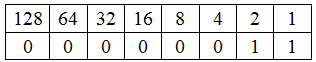## Python - Operators

Operators:

• Operators are symbols and used to perform some mathematics operations.
• There are 6 kinds of operators,
1.Arithmetic operators:
• This is normal mathematic operations such as,
 S.No Operator Expression Example Result 1. + Addition If a=10 and b=5 then a+b=10+5 15 2. - Subtraction If a=10 and b=5 then a-b=10-5 5 3. * Multiplication If a=10 and b=5 then a*b=10*5 50 4. / Division If a=10 and b=5 then a/b=10/5 2 5. % Modulo If a=10 and b=5 then a%b=10%5 0.05 6. ** Power If a=5 then a**2 25 7. // Floor division If a=10 and b=5 then a//b=7//5 1
Example program for the arithmetic operator:
1. a,b=input("Enter a and b value : ").split()
print("Add of "+a+" and "+b+" value is : ",int(a)+int(b))
print("Sub of "+a+" and "+b+" value is : ",int(a)-int(b))
print("Mul of "+a+" and "+b+" value is : ",int(a)*int(b))
print("Div of "+a+" and "+b+" value is : ",int(a)/int(b))
print("Mod of "+a+" and "+b+" value is : ",int(a)%int(b))
print("Floor divisions of "+a+" and "+b+" value is : ",int(a)//int(b))
print(a+" power 3 value is : ",int(a)**3)
• It is used to compare two or more data.
Note:
• These logical operators mostly used in conditions.
3. Logical Operators:
• It is used to combine simple relational statements into more complex expressions.
 S.No Operator Expression Example Result 1. and Both expressions should be true If  a=11,b=10 and c=12 then a>b and a>c False 2. or One expression should be true If  a=11,b=10 and c=12 then a>b or a>c True 3. not Both expression false then return true If  a=5,b=10 and c=12 then a>b not a>c True
4. Assignment Operators:
• These operators are used to getting the result in the same variable(input/output).
Example program for the assignment operator:
1. sal=int(input("Enter basic salary : "))

sal+=5000

print("Bouns with basic salary : ",sal)

Output:
5. Bitwise Operators:
• It is used to manipulate the data at a bit level and it operates on integer only.
Binary table

Bitwise AND(&):
• The truth table for ‘ & ‘is shown below(It means True=1 and false=0.)
a=7 of binary code:0111
b=8 of the binary code is:1000
The result of the a&a value is 0000(i.e 0).
Example program for the bitwise AND operator:
1. p,q=input("Enter two values : ").split()

r=int(p)&int(q)

print("Bitwise AND of p&q value is : ",r)

Output:
Bitwise OR(|):
• The truth table for ‘ | ‘is shown below
a=7 of binary code:0111
b=8 of the binary code is:1000
The result of a|b value is 1111(i.e 15).
Example program for the bitwise OR operator:
1. p,q=input("Enter two values : ").split()

r=int(p)|int(q)

print("Bitwise OR of p|q value is : ",r)

Output:
Bitwise Exclusive OR(^):
• The truth table for ‘ ^ ‘is shown below
a=13 of binary code:1101
b=8 of the binary code is:1000
The result of a^b value is 0101(i.e 5).
Example program for the bitwise Exclusive OR operator:
1. p,q=input("Enter two values : ").split()

r=int(p)^int(q)

print("Bitwise Exclusive-OR of p^q value is : ",r)

Output:
Bitwise Shift left (<<):
• The bitwise shift left operator is used to move the bits from right to left in storage.
• Each box called bits and a=6 and a<<1 then a=12.
Explanation:

1. a=int(input("Enter a values : "))

r=a<<1

print("Bitwise left shit of a value is: ",r)

Output:
Bitwise Shift right(>>):
• The shit right is similar to the left shit.
• This operator is used to forward the bits from left to right in storage.
• For example, If a=6 and a>>1 then its value is 3.

Explanation:

Result: a of right shit value is 3.Example program for the bitwise shift right operator:
1. a=int(input("Enter a values : "))

r=a>>1

print("Bitwise right shit of a value is: ",r)

Output:
Bitwise One's complement(~) or NOT:
• The truth table for ‘ ~ ‘is shown below
If a=6(binary code is 0110),
and ~a(0111) value is 7.
Using a negative signature for one's complement(~a=-7).
Example program for the bitwise one's complement operator:
1. p=int(input("Enter a values : "))

r=~p

print("Bitwise NOT of ~p value is: ",r)

Output:

6. Special operators:

Python language provides some special type of operators like identity operator and membership operator.

(i) Identity operator:

• An identity operator is used to checking both are equal or not equal.

 Operator Description Example Result is If both values are the same then return true. If a=4 and b=5 then a is b False is not If both values are not the same then return true. If a=4 and b=5 then a is not  b True

(ii) Membership operator:

• An identity operator is used to checking a given value is present in a list or words.

 Operator Description Example Result in If the values are present in the given list or anything then return true. If a=” Hello” then ‘l’ in a True not in If the values are not present not in the given list or anything then return true. If a=” Hello” then ‘i’ in a True
Example program for the special operator:
1. x=int(input("Enter x value: "))

y=int(input("Enter y value: "))

z=input("Enter any word: ")

print("x is equal to y: ",x is y)

print("x is not equal to y: ",x is not y)

print("Character \'a\' in "+z+" : ",'a' in z)

print("Character \'p\' not in "+z+" : ",'a' not in z)

Output: